21 Graphs and Networks
A very simple graph of connections:
 In:=Out=In:=Out=In:=Out=Add two more connections, including one connecting 2 right back to 2:
 In:=Out=In:=Out=The shortest path from 4 to 2 on the graph goes through 1:
 In:=Out=Now lets make another graph. This time lets have 3 nodes, and lets have a connection between every one of them.
Start by making an array of all possible connections between 3 objects:
 In:=Out=The result here is a list of lists. But what Graph needs is just a single list of connections. We can get that by using Flatten to flatten out the sublists.
Flatten flattens out all sublists, wherever they appear:
 In:=Out=Get a flattened list of connections from the array:
 In:=Out=In:=Out=In:=Out=In:=Out=Make a graph with 20 connections between randomly chosen nodes numbered from 0 to 10:
 In:=Out=Youll get a different graph if you generate different random numbers. Here are 6 graphs.
Six randomly generated graphs:
 In:=Out=In:=Out=The result is a graph with the exact same connections as the original, but with the nodes arranged to illustrate the community structure of the graph.
21.1Make a graph consisting of a loop of 3 nodes. »
Expected output:
 Out[]=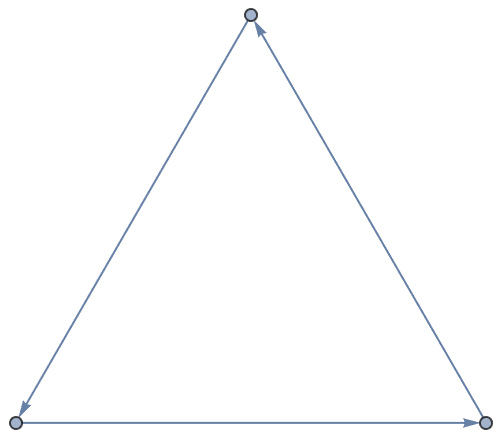Answer & check your solution
21.2Make a graph with 4 nodes in which every node is connected. »
Expected output:
 Out[]=Answer & check your solution
21.3Make a table of undirected graphs with between 2 and 10 nodes in which every node is connected. »
Expected output:
 Out[]=Answer & check your solution
21.4Use Table and Flatten to get {1, 2, 1, 2, 1, 2}»
Expected output:
 Out[]=Answer & check your solution
21.5Make a line plot of the result of concatenating all digits of all integers from 1 to 100 (i.e. ..., 8, 9, 1, 0, 1, 1, 1, 2, ...). »
Expected output:
 Out[]=Answer & check your solution
21.6Make a graph with 50 nodes, in which node i connects to node i+1»
Expected output:
 Out[]=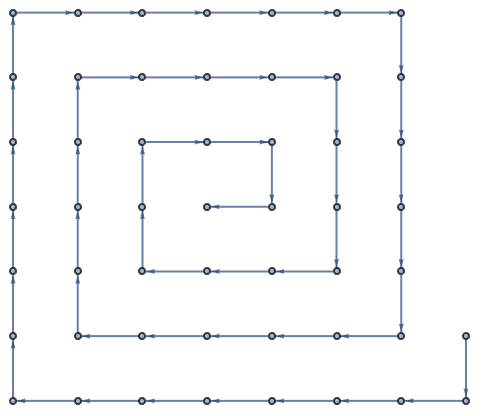Answer & check your solution
21.7Make a graph with 4 nodes, in which each connection connects i to Max[i, j]»
Expected output:
 Out[]=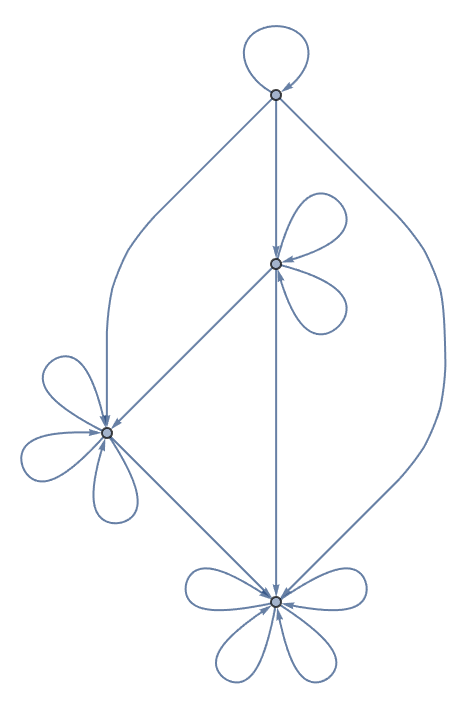Answer & check your solution
21.8Make a graph in which each connection connects i to j-i, where i and j both range from 1 to 5. »
Expected output:
 Out[]=Answer & check your solution
21.9Generate a graph with 100 nodes, each with a connection going to one randomly chosen node. »
Sample expected output:
 Out[]=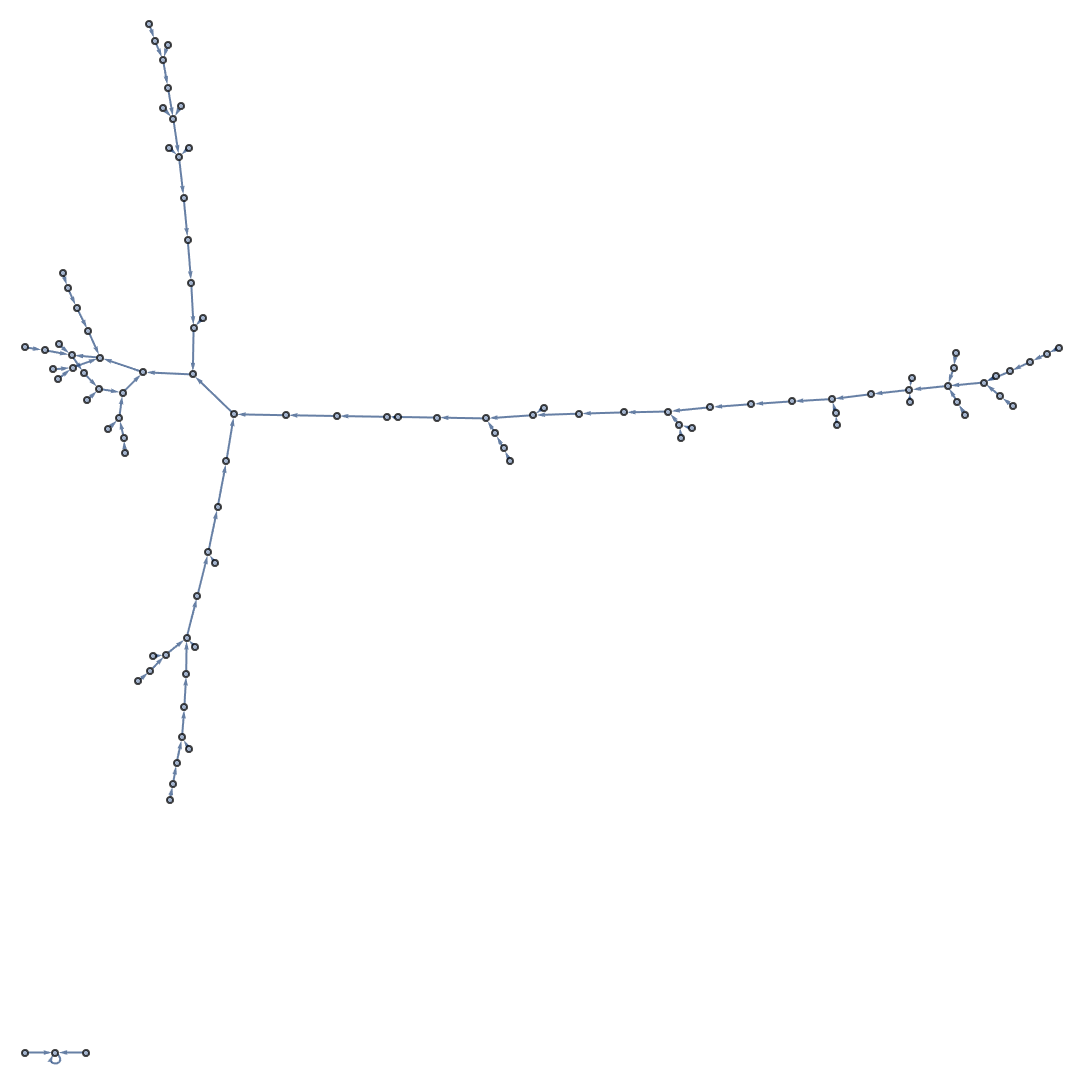Answer & check your solution
21.10Generate a graph with 100 nodes, each connecting to two randomly chosen nodes. »
Sample expected output:
 Out[]=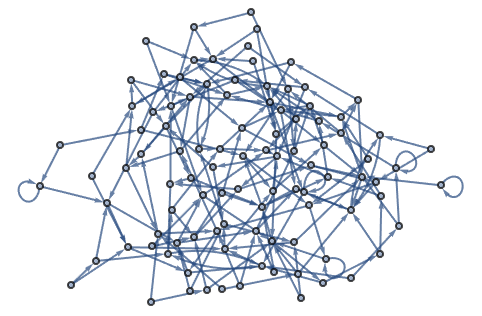Answer & check your solution
21.11For the graph {12, 23, 34, 41, 31, 22}, make a grid giving the shortest paths between every pair of nodes, with the start node as row and end node as column. »
Expected output:
 Out[]=Answer & check your solution
+21.1Make a graph with 4 nodes in which every node is connected, displaying the resulting graph with radial drawing. »
Expected output:
 Out[]=Answer & check your solution
+21.2Generate a graph in which a single node is connected to 10 others. »
Expected output:
 Out[]=Answer & check your solution
+21.3Use Flatten to generate a table of the numbers 1 to 50 in which even numbers are colored red. »
Expected output:
 Out[]=Answer & check your solution
+21.4Use ImageData, Flatten and Total to find the number of white pixels in Binarize[Rasterize["W"]]»
Expected output:
 Out[]=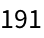Answer & check your solution
+21.5Use Flatten, IntegerDigits and Total to find the sum of all digits of all whole numbers up to 1000. »
Expected output:
 Out[]=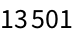Answer & check your solution
+21.6Generate a graph with 200 connections, each between nodes with numbers randomly chosen between 0 and 100. »
Sample expected output:
 Out[]=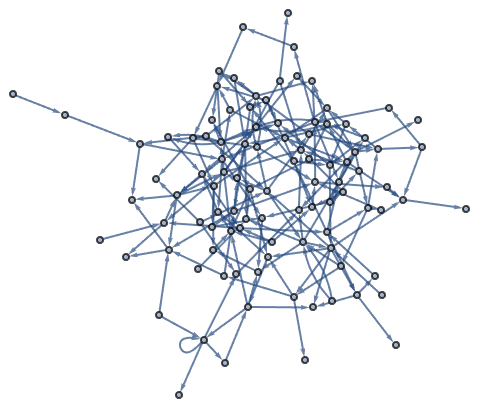Answer & check your solution
+21.7Generate a plot that shows communities in a random graph with nodes numbered between 0 and 100, and 200 connections. »
Sample expected output:
 Out[]=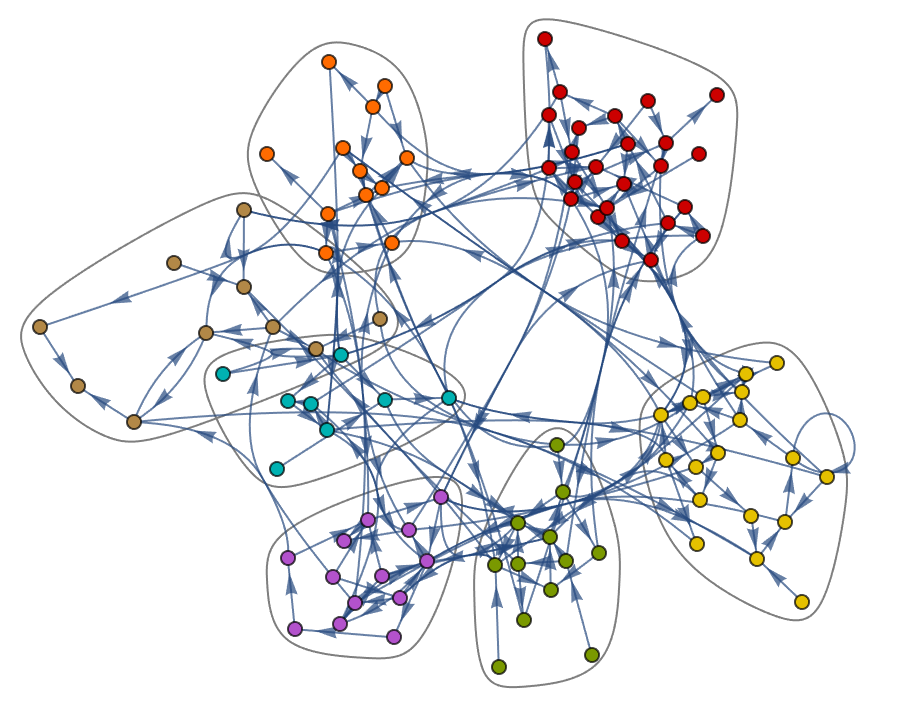Answer & check your solution
Whats the difference between a graph and a network?
Theres no difference. Theyre just different words for the same thing, though graph tends to be more common in math and other formal areas, and network more common in more applied areas.
Vertices are the points, or nodes, of a graph. Edges are the connections. Because graphs have arisen in so many different places, there are quite a few different names used for the same thing.
How is ij understood?
Its Rule[i, j]. Rules are used in lots of places in the Wolfram Languagesuch as giving settings for options.